Finding Area by Changing units

Chapter 10 Class 6 Mensuration
Concept wise

We have learnt how to change units like mm to cm, or cm to km

Let’s learn how to change square units as well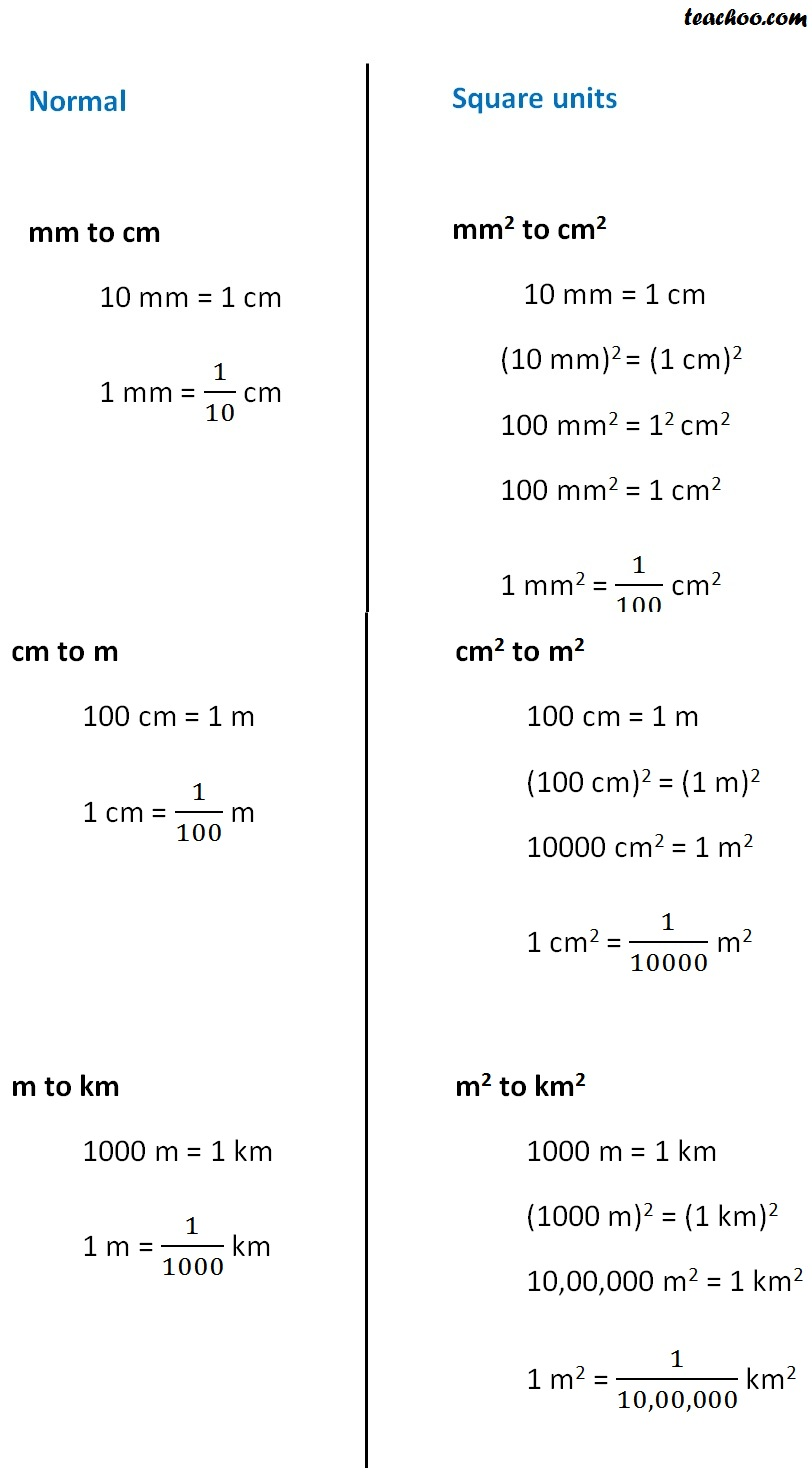## Hectares

Hectare is also a unit used to measure area.

1 hectare actually means 1 hecto metre square

1 hectare = 1 hector metre 2

1 hectare = 1 hm 2

Also,

1 hm = 100 m

(1 hm) 2 = (100 m) 2

1 hm 2 = 100 × 100 m 2

1 hm 2 = 10,000 m 2

∴ 1 hectare = 10,000 m 2

Lets take some examples

## Find area of rectangle in m 2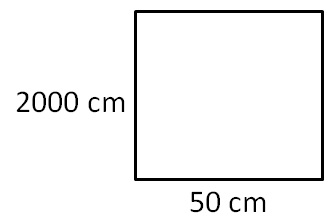Length = l = 50 cm

Breadth = b = 2000 cm

Area = l × b

= 50 × 2000

= 1,00,000 cm 2

= 1,00,000 × (1/100) 2 m 2

= 1,00,000 × 1/10,000 m 2

= 10 m 2

## Find area of rectangle in cm 2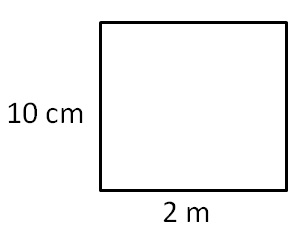Length = l = 2 m

= 2 × 100 cm

= 200 cm

Breadth = b = 10 cm

Area = l × b

= 200 × 10

= 2000 sq. cm

= 2000 cm 2

## Find area in m 2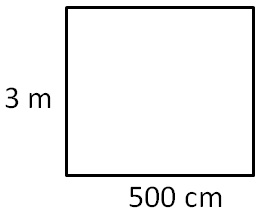Length = l = 500 cm

= 500 × 1/100 cm (As 1 cm = 1/100 m)

= 5 m

Breadth = b = 3 m

Area = l × b

= 5 × 3

= 15 sq. m

= 15 m 2

## Find area of rectangle in m 2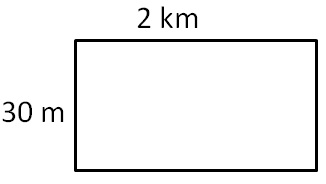Length = l = 2 km

= 2 × 1000 m       (1 km = 1000 m)

= 2000 m

Breadth = b = 30 m

Area = l × b

= 2,000 × 30

= 60,000 sq. m

= 60,000 m 2

## Find area of rectangle in km 2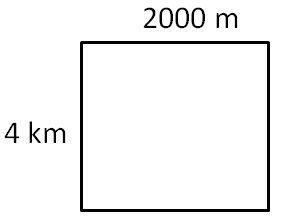Length = l = 2000 m

= 2000 × 1/1000 km           (1m = 1/1000 km )

= 2 km

Breadth = b = 4 km

Area = l × b

= 2 × 4

= 8 sq. km

= 8 km 2

## Find area of rectangle in hectare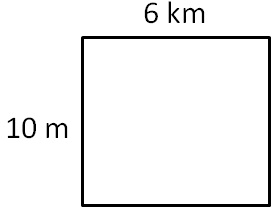Length = l = 6 km

= 6 × 1000 m     (1km = 1000 m)

= 6000 m

Breadth = b = 10 m

Area = l × b

= 6000 × 10

= 60,000 m 2

= 60,000 × 1/10,000 hectare    (1m 2 = 1/10,000  hectare)

= 6 hectare

## Find area of rectangle in hectare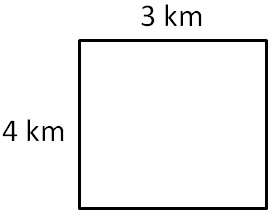Length = l = 3 km

Breadth = b = 4 km

Area = l × b

= 3 × 4

= 12 km 2

= 12 × 10,00,000 m 2                                             (1km 2 = 10,00,000 m)

= 1,20,00,000 m

= 1,20,00,000 × 1/10,000 hectare           (1m 2 = 1/10,000  hectare)

= 1,200 hectare

## Change units

### 50 cm 2  in mm 2

= 50 cm 2

= 50 × 1 cm 2

= 50 × (10 mm) 2               (1 cm = 10 mm)

= 50 × 100 mm 2

= 5,000 mm 2

### 2 ha  in m 2

= 2 hectare

= 2 × 10,000 m 2         (1ha = 10,000 m 2 )

= 20,000 m 2

### 10 m 2  in cm 2

= 10 m 2

= 10 × 1 m 2

= 10 × (100 cm) 2

= 10 × 10,000 cm 2         (1 m = 100 cm)

= 1,00,000 cm 2

### 10 cm 2  in m 2

= 10 cm 2

= 10 × 1 cm 2

= 10 × (1/100  m) 2

= 10 × 1/10000 m 2                       (1 cm = 1/100 m)

= 1/1000 m 2

= 0.001 m 2

Get live Maths 1-on-1 Classs - Class 6 to 12

### Transcript

Normal mm to cm 10 mm = 1 cm 1 mm = 1/10 cm Square units mm2 to cm2 10 mm = 1 cm (10 mm)2 = (1 cm)2 100 mm2 = 12 cm2 100 mm2 = 1 cm2 1 mm2 = 1/100 cm2 cm to m 100 cm = 1 m 1 cm = 1/100 m m to km 1000 m = 1 km 1 m = 1/1000 km cm2 to m2 100 cm = 1 m (100 cm)2 = (1 m)2 10000 cm2 = 1 m2 1 cm2 = 1/10000 m2 m2 to km2 1000 m = 1 km (1000 m)2 = (1 km)2 10,00,000 m2 = 1 km2 1 m2 = 1/10,00,000 km2